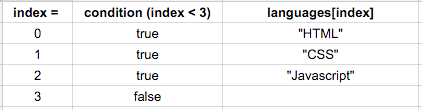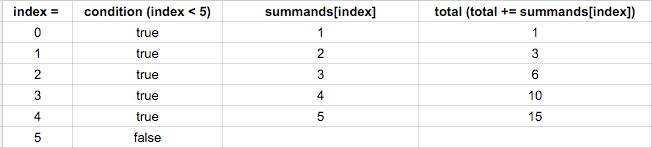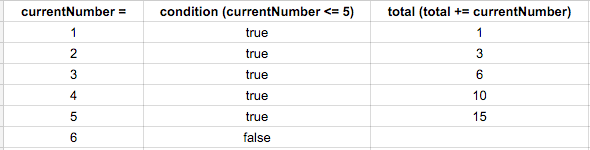`forEach()` is a nice, easy-to-read, easy-to-understand method, but it's actually a pretty new addition to JavaScript. It also is limited for use on collections of information where processing iterates through each element in the collection. Let's take a look at a more traditional way of looping called a for loop.

``````for (var index = 1; index <= 3; index += 1) {
}
``````

Here's how the `for` loop works:

• The `for` statement takes three parameters: initialization, condition, and final expression.
• The initialization parameter lets you initialize a variable at the start of the for loop. In this case, we've initialized a variable called `index` that starts at 1. This is the starting place for the loop. The initialization parameter is executed before the loop runs.
• The condition parameter tells the loop when to stop running - or more accurately when to keep running. The loop will stop when the condition is no longer true. In our example, when the index variable is NOT less than or equal to 3, the loop will stop. Until then, it will keep going. The condition is evaluated every time the loop runs.
• The final expression parameter usually changes the initial value in some way; often by incrementing or decrementing it. In our example, the index variable will be increased by 1 after each time the loop is executed.
• Each time the loop runs, the code within the loop will pop up an alert with the number of the pass we're on (index).

Here's an example we saw in the last lesson re-written using a `for` loop instead of a `forEach` loop:

``````var languages = ['HTML', 'CSS', 'Javascript'];
for (var index = 0; index < languages.length; index += 1) {
alert('I love ' + languages[index] + '!');
}
``````

There is a new concept in this loop: `languages.length`. `length` is not a method. Notice it's missing the parentheses at the end. Length is a property on every array we create. Strings also have this property, e.g. `'foobar'.length`.

``````> var languages = ['HTML', 'CSS', 'Javascript'];
> languages.length;
3

var school = "epicodus";
school.length;
8
``````

When we explore objects next week, we will talk more about properties but for now, let's continue looping.

In the for loop example above, we have an array containing 3 elements. When we first start the loop, we run our initialization statement (`var index = 0`), which creates a variable called `index` and initializes it with the value `0`. We are now done with initialization, which only happens once, when we first enter the for loop. From now on the flow is:

1. check condition: `index < languages.length` (continue to step 2 only if condition evaluates to `true`, otherwise exit out of the for loop)
2. execute code block: `alert('I love ' + languages[index] + '!');`
3. execute "final expression" update statement: `index += 1`
4. repeat, beginning with step 1 above

The first time through the loop index is 0, so languages[index] is "HTML". The second time through the loop index is 1, so languages[index] is "CSS". The third time through the loop index is 2, so languages[index] is "Javascript". The fourth time we try to enter the loop, index is 3 which is not less than `languages.length` (3), so we don't enter the loop again.

Here's a chart that may help illustrate the loop flow:We previously used a `forEach()` loop to add all the elements of an array together. Let's rewrite that one too with a `for` loop:

``````var total = 0;
var summands = [1, 2, 3, 4, 5];
for (var index = 0; index < summands.length; index += 1) {
total += summands[index];
}
``````

Here's a corresponding chart:Note that when index is 0 here, summands[index] is 1 because the first element of the array is 1. index is just being used here to refer to which element of the array - it's a bit confusing in this case because the elements of the array are also numbers.

The `forEach()` method would have worked just as well in those above cases, but sometimes you'll find yourself in a situations where you need a regular `for` loop rather than a `forEach()` loop - for instance when you need to run a loop a certain number of times, rather than looping over an array.

Here's a version of our sum code that uses a `for` loop but doesn't require an array to loop through:

``````var total = 0;
for (var currentNumber = 1; currentNumber <= 5; currentNumber += 1) {
total += currentNumber;
}
``````

When the loop begins we initialize our loop variable currentNumber to 1. (Note that we could have used index as the variable name here, but currentNumber seems clearer since we'll be making use of the variable for something other than iterating through a loop; we'll be adding it to total each time through the loop in addition to using it as an iterator.) We then execute our loop as long as currentNumber is less than or equal to 5, iterating currentNumber after each run through the loop. Here's a chart showing the steps:The first time through the loop, `currentNumber` is 1, which is <= 5, so we go ahead and execute the statement in the code block: `total += currentNumber`. `total` is now 1 after this first time through the loop. At the end of each time through the loop we're incrementing `currentNumber`, so `currentNumber` is now 2.

The second time through the loop, `currentNumber` is 2, which is still <= 5, so again we execute `total += currentNumber`, setting total to 3. We again increment `currentNumber`.

The third time through, `currentNumber` is 3, so total now becomes 6. And we increment `currentNumber`.

The fourth time through, `currentNumber` is 4, so total becomes 10 and then we increment `currentNumber`.

The fifth time through, `currentNumber` is 5, so total becomes 15 and we increment `currentNumber`.

The sixth time we try to start going through the loop, `currentNumber` is 6, which is not <= 5, so we're done with the for loop. We then show the user an alert that includes the total.

Example `for` loop:

``````for (var index = 1; index <= 3; index += 1) {
Example of using a `for` loop with an array:
``````var languages = ['HTML', 'CSS', 'Javascript'];